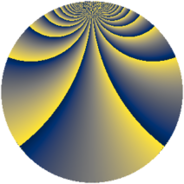# Properties

 Label 684.3.ciLevel $684$ Weight $3$ Character orbit 684.ci Rep. character $\chi_{684}(59,\cdot)$ Character field $\Q(\zeta_{18})$ Dimension $1416$ Sturm bound $360$

# Related objects

## Defining parameters

 Level: $$N$$ $$=$$ $$684 = 2^{2} \cdot 3^{2} \cdot 19$$ Weight: $$k$$ $$=$$ $$3$$ Character orbit: $$[\chi]$$ $$=$$ 684.ci (of order $$18$$ and degree $$6$$) Character conductor: $$\operatorname{cond}(\chi)$$ $$=$$ $$684$$ Character field: $$\Q(\zeta_{18})$$ Sturm bound: $$360$$

## Dimensions

The following table gives the dimensions of various subspaces of $$M_{3}(684, [\chi])$$.

Total New Old
Modular forms 1464 1464 0
Cusp forms 1416 1416 0
Eisenstein series 48 48 0

## Trace form

 $$1416q - 9q^{2} - 3q^{4} - 18q^{5} - 6q^{6} - 36q^{9} + O(q^{10})$$ $$1416q - 9q^{2} - 3q^{4} - 18q^{5} - 6q^{6} - 36q^{9} - 12q^{10} - 9q^{12} - 6q^{13} - 9q^{14} - 3q^{16} - 18q^{20} - 66q^{21} - 51q^{22} - 138q^{24} - 6q^{25} - 216q^{26} + 36q^{28} - 18q^{29} - 417q^{30} - 9q^{32} - 39q^{34} + 54q^{36} - 9q^{38} + 147q^{40} - 18q^{41} + 3q^{42} + 153q^{44} - 6q^{45} - 18q^{46} + 60q^{48} + 4458q^{49} - 18q^{50} - 3q^{52} - 213q^{54} - 441q^{56} - 12q^{57} - 6q^{58} - 675q^{60} - 6q^{61} - 36q^{62} - 204q^{64} - 36q^{65} + 438q^{66} - 9q^{68} - 18q^{69} - 297q^{70} + 228q^{72} - 96q^{73} - 351q^{74} - 3q^{76} - 36q^{77} - 747q^{78} + 828q^{81} - 12q^{82} - 9q^{84} - 6q^{85} - 495q^{86} - 9q^{88} - 213q^{90} - 9q^{92} - 120q^{93} + 447q^{96} - 366q^{97} - 405q^{98} + O(q^{100})$$

## Decomposition of $$S_{3}^{\mathrm{new}}(684, [\chi])$$ into newform subspaces

The newforms in this space have not yet been added to the LMFDB.